# GRE Geometry - Triangles

When it comes to geometry, triangles become the most favorite topic of standardized exams and GRE is no exception. Triangles is a very important topic in the GRE Quantitative Reasoning section. Therefore it is crucial to understand its concepts and properties.

So what is a triangle and what are its properties?

Triangle is the simplest form of a polygon and is made up of just three sides. It is essential to remember that the sum of all the internal angles of a triangle is equal to 180 degrees.

## Types of Triangles

Triangles can be categorized based on different attributes. Let us look at some of the attributes on which we can divide the triangles.

### Based on Side Length

1. Equilateral Triangle: All sides, as well as all angles of an equilateral triangle, are equal. Hence, $Area \; of \; Equilateral \; Triangle = \frac{\sqrt{3}}{4}a^{2}$, where ‘a’ is the length of the side of equilateral triangle. Each angle of an equilateral triangle is equal to 60°.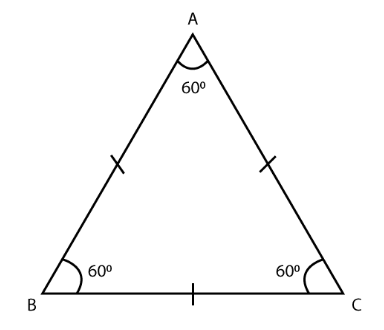1. Isosceles Triangle: Two of the sides, as well as two angles of an isosceles triangle, are equal.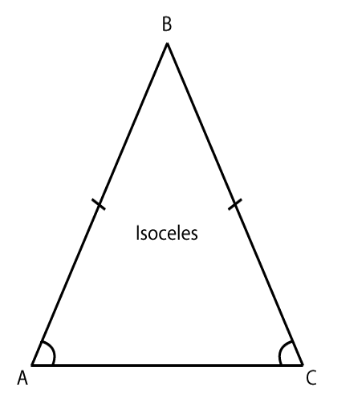1. Scalene Triangle: Neither any side nor any angles are equal in a scalene triangle.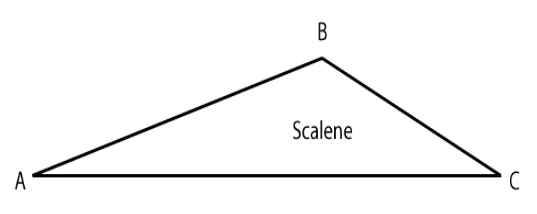### Based on Angles

1. Acute Triangle: All the three angles in an acute angled triangle is less than 90°.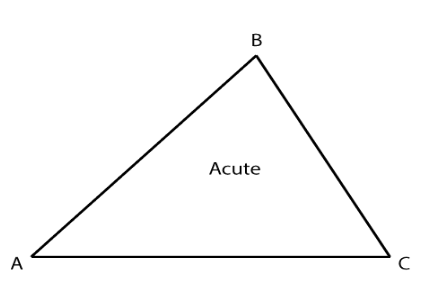1. Right Angle Triangle: If one of the angles of the triangle is equal to 90 degrees, such a triangle is known as right angled triangle.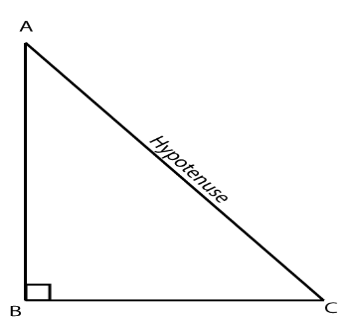1. Obtuse Triangle: In an obtuse angled triangle, one of the angles in the triangle is greater than 90°.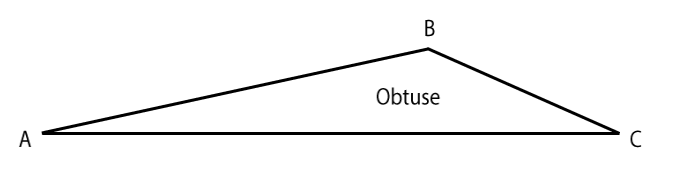### Based on Length & Angle

1. Right Isosceles Triangle: It is a combination of both isosceles as well as right angled triangle. So it holds all the properties of both isosceles as well as right angled triangle.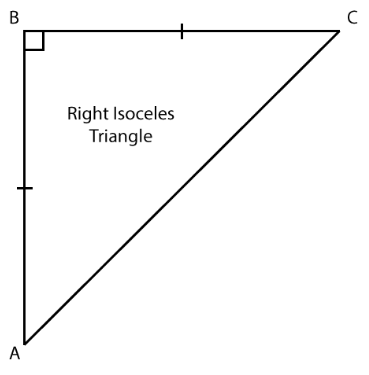1. Obtuse Isosceles Triangle: It is a combination of both isosceles as well as obtuse angled triangle. Hence, it holds all the properties of both isosceles as well as obtuse angled triangle.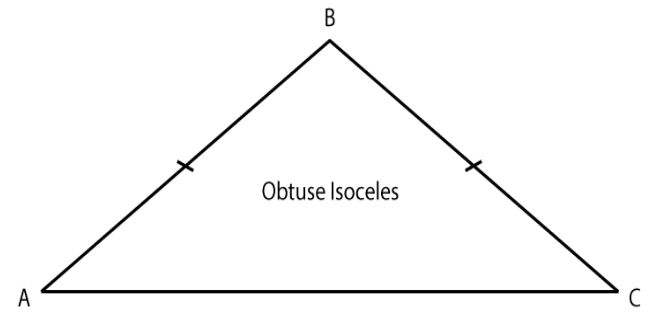## Properties of Similar Triangles

• The corresponding angles of similar triangles are the same.
• The corresponding sides of similar triangles are in the same proportion.
• The corresponding medians of similar triangles are all in the same ratio.
• The corresponding altitudes of similar triangles are all in the same ratio.
• The ratio of the areas is the square of the ratio of the sides of similar triangle. For example, if the side lengths of triangle A is 4, 5, and 6 inches, and the side length of triangle B is 8, 10, and 12 inches, then the ratio of the sides of these triangles is 2:1, hence, the ratio of their areas will be $(2)^{2}:(1)^{2} = 4:1$

BYJU’S will be glad to help you in your GRE preparation journey. You can ask for any assistance related to GRE from us by just giving a missed call at +918884544444, or you can drop an SMS. You can write to us at gre@byjus.com